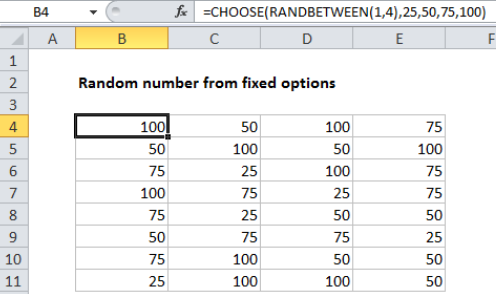## Excel Office

Excel How Tos, Tutorials, Tips & Tricks, Shortcuts

# How to fill cell ranges with random number from fixed set of options in Excel

To quickly fill a range of cells with random numbers from a set of fixed options (i.e. 25,50,75,100), you can use a formula based on the CHOOSE and RANDBETWEEN functions.

## Formula

`=CHOOSE(RANDBETWEEN(1,4),num1,num2,num3,num4)`## Explanation

In the example shown, the formula in B4 is:

`=CHOOSE(RANDBETWEEN(1,4),25,50,75,100)`

Which returns a random number from the numbers provided.

### How this formula works

The CHOOSE function does most of the work in this formula. Choose takes a single numeric value as its first argument (index_number), and uses this number to select and return one of the values provides as subsequent arguments, based on their numeric index.

Worked Example:   Excel VLOOKUP and HLOOKUP functions Examples

In this case, we are providing four numbers as options: 25,50,75,100, so we need to give CHOOSE a number between 1 and 4.

Worked Example:   Get first Monday before any date in Excel

To generate this number, we use RANDBETWEEN, a function that returns a random integer based on a lower and upper bound. Since we are only working with 4 values in CHOOSE, we supply 1 for the bottom number and 4 for the top number.

When this formula is copied down, it will return one of the four numbers.

Worked Example:   How to get Holiday Date from Year in Excel

Note that RANDBETWEEN will calculate a new value whenever the worksheet is changed. Once you have values in the range, you may want to replace the formulas with values to prevent further changes. You can do this with paste special: select all formulas, copy, the paste special > values to replace formulas with values.